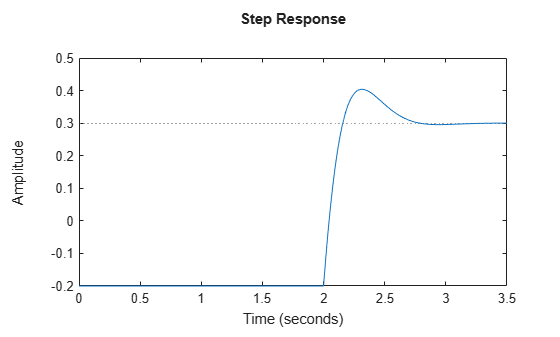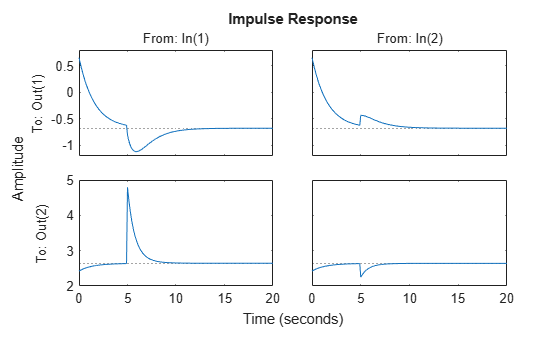# RespConfig

Options for step or impulse responses

Since R2023a

## Description

Use a `RespConfig` object to specify options for the `step` and `impulse` responses.

The `step` function applies this configuration as follows.

• In the SISO case

`$u\left(t\right)=\left\{\begin{array}{cc}U,& t<{T}_{0}+{T}_{d}\\ U+dU,& t\ge {T}_{0}+{T}_{d}.\end{array}$`
• In the MIMO case, the step response of the jth input channel is obtained by setting

`$u\left(t\right)=\left\{\begin{array}{cc}U,& t<{T}_{0}+{T}_{d}\\ U+d{U}_{j}*{e}_{j},& t\ge {T}_{0}+{T}_{d}\end{array},$`

where ej is the jth basis vector.

The `impulse` function applies this configuration as follows.

`$u\left(t\right)=U+dU*\delta \left(t-\left({T}_{0}+{T}_{d}\right)\right)$`

Here:

• U is the input signal offset.

• dU is the input level change relative to U.

• T0 is the start time.

• Td is the time at which the change occurs relative to T0.

## Creation

### Syntax

``respOpt = RespConfig``
``respOpt = RespConfig(Name=Value)``

### Description

example

````respOpt = RespConfig` creates a response configuration object with default property values.```

example

````respOpt = RespConfig(Name=Value)` creates a response configuration object and sets its properties using one or more name-value arguments.```

## Properties

expand all

Input signal offset level, specified as a scalar or vector.

• For single-input systems, `InputOffset` is a scalar value.

• For multi-input systems, `InputOffset` is a vector of length Nu, where Nu is the number of input channels. Each vector value corresponds to the signal offset in that input channel. The functions compute the responses one input channel at a time.

• For linear parameter-varying (LPV) and linear time-varying (LTV) models, use a scalar or vector to specify a constant offset. Otherwise, set `InputOffset` = `'u0'` to apply the step or impulse change relative to the input offsets u0(t) of the LTV model or u0(t,p) of the LPV model. This is the `u0` output of the data function. The total input signal is then u0 + u(t).

Input level change relative to the input signal offset, specified as a scalar or vector.

• For single-input systems, `Amplitude` is a scalar value.

• For multi-input systems, `Amplitude` is a vector of length Nu, where Nu is the number of input channels. Each vector value corresponds to the level change amplitude in that input channel. The functions compute the responses one input channel at a time.

Input signal delay, specified as a nonnegative scalar value. This value specifies when the change occurs relative to the simulation start time T0.

Initial state values, specified as a vector or `initialCondition` object.

By default, the simulation starts from the steady-state condition obtained for a constant input level equal to the input offset U. For state-space models without internal delays, the initial state xinit is obtained by solving:

• Continuous time — 0 = Axinit + BU

• Discrete time — xinit = Axinit + BU

To start the simulation from a different initial state, use one of the following.

• For state-space models, `InitialState` is a vector of length Nx, where Nx is the number of states.

• For LTV and LPV models, you can also set `InitialState` to `'x0'`. This uses the state offset value x0(T0) or x0(T0,p(T0)). The state offset value is the `x0` output of the data function evaluated at t = T0.

• For identified LTI models, such as `idtf`, `idss`, `idproc`, `idpoly`, and `idgrey` models, you can use an `initialCondition` object to specify the initial state. Using identified models requires System Identification Toolbox™ software.

Initial parameter values for LPV models, specified as a scalar or vector.

This property is required only when you specify `InputOffset` = `'u0'` or `InitialState` = `[]` or `'x0'`. Otherwise, this value is ignored.

## Object Functions

 `impulse` Impulse response plot of dynamic system; impulse response data `step` Step response of dynamic system

## Examples

collapse all

Create a transfer function model.

`sys = tf([1 5],[1 10 50]);`

Create an option set to specify step input offset, amplitude, and delay.

`Config = RespConfig('InputOffset',-2,'Amplitude',5,'Delay',2);`

Calculate the step response using the specified options.

`step(sys,Config)`Create a state-space model.

```A = [-0.8429,-0.2134;-0.5162,-1.2139]; B = [0.7254,0.7147;0,-0.2050]; C = [-0.1241,1.4090;1.4897,1.4172]; D = [0.6715,0.7172;-1.2075,0]; sys = ss(A,B,C,D);```

Create a default option set and use the dot notation to specify values.

```respOpt = RespConfig; respOpt.InputOffset = [-2,3]; respOpt.Amplitude = [2,-0.5]; respOpt.InitialState = [0.1,-0.1]; respOpt.Delay = 5;```

Compute the impulse response.

```t = 0:0.1:20; impulse(sys,t,respOpt)```## Version History

Introduced in R2023a

expand all